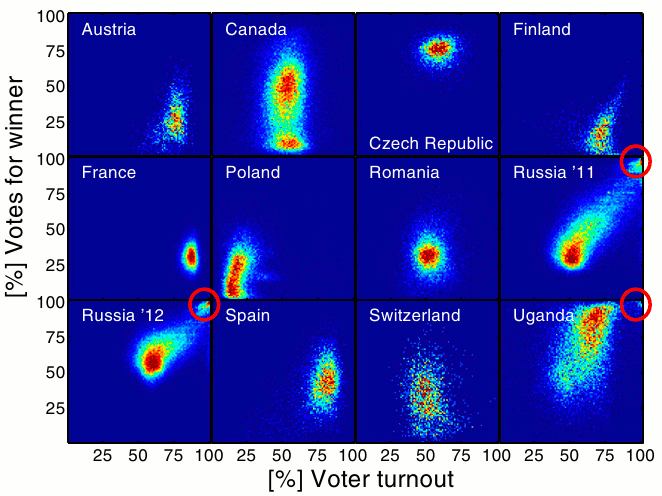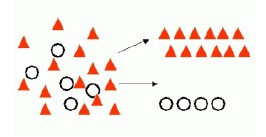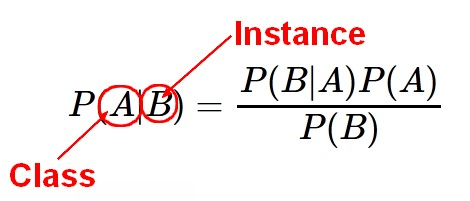# Data Mining - (Class|Category|Label) Target

A class is the category for a classifier which is given by the target. The number of class to be predicted define the classification problem.

A class is also known as a label.

## Spark

``````from pyspark.mllib.regression import LabeledPoint

firstLabeledPoint = LabeledPoint('Play',[1,2,3])
SecondLabeledPoint = LabeledPoint('Don''t Play',[2,2,3])

firstLabeledPoint.label
firstLabeledPoint.features```
```

Discover More(Machine|Statistical) Learning - (Target|Learned|Outcome|Dependent|Response) (Attribute|Variable) (Y|DV)

An (outcome|dependent) variable is ameasure that we want to predict. : the original score collected : the predicted score (or estimator) from the equation. The hat means “estimated” from the...Data Mining - (Anomaly|outlier) Detection

The goal of anomaly detection is to identify unusual or suspicious cases based on deviation from the norm within data that is seemingly homogeneous. Anomaly detection is an important tool: in data...Data Mining - (Classifier|Classification Function)

A classifier is a Supervised function (machine learning tool) where the learned (target) attribute is categorical (“nominal”) in order to classify. It is used after the learning process to classify...Data Mining - (Function|Model)

The model is the function, equation, algorithm that predicts an outcome value from one of several predictors. During the training process, the models are build. A model uses a logic and one of several...Data Mining - (two class|binary) classification problem (yes/no, false/true)

Binary classification is used to predict one of two possible outcomes. A two class problem (binary problem) has possibly only two outcomes: “yes or no” “success” or “failure” and is...Data Mining - Entropy (Information Gain)

The degree to which a system has no pattern is known as entropy. A high-entropy source is completely chaotic, is unpredictable, and is called true randomness. Entropy is a function “Information”...Data Mining - Maximum Entropy Algorithm

Maximum Entropy (MaxEnt) models are feature-based classifier models. In a two-class scenario, it is the same as using logistic regression to find a distribution over the classes. MaxEnt makes no...Data Mining - Multi-class (classification|problem)

Multiclass classification is used to predict: one of three or more possible outcomes and the likelihood of each one. Generally, there is no notion of closeness because the target class is nominal....Data Mining - Naive Bayes (NB)

Naive Bayes (NB) is a simple supervised function and is special form of discriminant analysis. It's a generative model and therefore returns probabilities. It's the opposite classification strategy...Data Mining - Problem

A page the problem definition in data Type of target: nominal or quantitative Type of target class: binomial of multiclass Number of parameters: Type of (predictor|features): nominal or numeric....